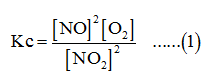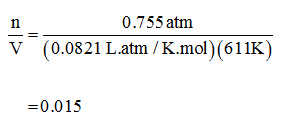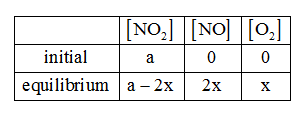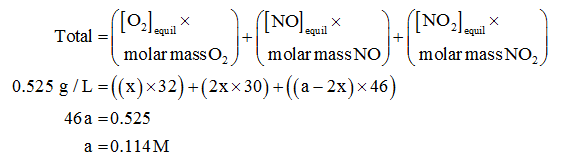# A sample of pure NO2 is heated to 338 ∘C at which temperature it partially dissociates according to the equation2NO2(g)⇌2NO(g)+O2(g)At equilibrium the density of the gas mixture is 0.525 g/L at 0.755 atm. Calculate Kc for the reaction

Question
27 views

A sample of pure NO2 is heated to 338 ∘C at which temperature it partially dissociates according to the equation
2NO2(g)⇌2NO(g)+O2(g)
At equilibrium the density of the gas mixture is 0.525 g/L at 0.755 atm. Calculate Kc for the reaction

check_circle

Step 1

The density of gas mixture = 0.525 g/L

The pressure is 0.755 atm

The dissociation of pure NO2 is,

2NO2(g)⇌2NO(g)+O2(g)

The equilibrium constant for the reaction is,The ideal gas equation at equilibrium is,

PV=nRT

The temperature is 611K.

The value of R=0.0821 L.atm/K.mol

On substituting the values in ideal gas equation,Step 2

The ice table for the equation (1) is given below.The total mass of mixture at equilibrium is calculated by the expression,...

### Want to see the full answer?

See Solution

#### Want to see this answer and more?

Solutions are written by subject experts who are available 24/7. Questions are typically answered within 1 hour.*

See Solution
*Response times may vary by subject and question.
Tagged in